# TP1: Canny Edges¶

To execute a notebook, you will need to install jupyter. If you are using anaconda (strongly advised). If you cannot/don't want to use notebooks, you can return both your python code and a report in pdf. Note that all the TPs will use python2. If you are using python3, you might need to do a few changes to the provided code.

Return your work by e-mail using a single file (ipynb or zip) with the format 'introvis17_tp1_yourname.ipynb'

The first part is about doing basic image processing in Python, the second breaks down the different steps in implementing the Canny edge detector.

## 1. Basic image processing in Python¶

Here are a set of useful libraries for image processing. You will need to refer to the online documentation of the different libraries to complete the TP.

In :
import numpy as np
# this is the key library for manipulating arrays. Use the online ressources! http://www.numpy.org/

import matplotlib.pyplot as plt
# used to read images, display and plot http://matplotlib.org/api/pyplot_api.html .
# You can also check this simple intro to using ipython notebook with images
# https://matplotlib.org/users/image_tutorial.html .

%matplotlib inline
# to display directly in the notebook

import scipy.ndimage as ndimage
# one of several python libraries for image procession

plt.rcParams['image.cmap'] = 'gray'
# by default, the grayscale images are displayed with the jet colormap: use grayscale instead


Here is a simple example how to read and display an image (you need to first download this image)

In :
name='lena.jpg'
plt.imshow(I)

Out:
<matplotlib.image.AxesImage at 0x1814292320>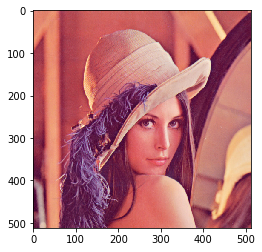### a- What's the size of the image I? Its type? The type of the values it contains? What's its range?¶

In :
# complete this part.
# Tip: look for numpy attributes (e.g. https://docs.scipy.org/doc/numpy/reference/arrays.ndarray.html)
# and functions to do what you want (e.g. np.min)

# /!\: I will resort to PYTHON3
from IPython.display import display, Markdown

# data type range of numpy dtypes
dtype_range = {np.bool_.__name__: (False, True),
np.bool8.__name__: (False, True),
np.uint8.__name__: (0, 255),
np.uint16.__name__: (0, 65535),
np.int8.__name__: (-128, 127),
np.int16.__name__: (-32768, 32767),
np.int64.__name__: (-2**63, 2**63 - 1),
np.uint64.__name__: (0, 2**64 - 1),
np.int32.__name__: (-2**31, 2**31 - 1),
np.uint32.__name__: (0, 2**32 - 1),
np.float32.__name__: (-1, 1),
np.float64.__name__: (-1, 1)}

display(Markdown("- **Size of I:** {} \n"\
"- Its *type*: {} \n"\
"- The **type of its values**: {} \n"\
"    - whose **range** is: {} \n"\
"- Its **shape**: {}"\
.format(I.size, type(I).__name__, I.dtype, dtype_range[str(I.dtype)], I.shape)))

• Size of I: 786432
• Its type: ndarray
• The type of its values: uint8
• whose range is: (0, 255)
• Its shape: (512, 512, 3)

### b- Here is a simple function to load an image and crop it according to a bounding box. Make sure you understand every line.¶

In :
def load_image_v0(name,crop_window=-1):
if crop_window!=-1:
I=I[crop_window:crop_window,crop_window:crop_window] # slices the image according to crop_window
I=I.astype('float')/255
return I


### What is the line I=I.astype('float') doing? Use the function to extract and save the 100x50 pixels of the bottom left corner of 'lena'.¶

The line I=I.astype('float') casts the values of I to the type float, so that the division performed afterwards is a floating point one, and the resulting float image is restricted to the range (-1, 1).

In :
dh, dw = 100, 50 # size of the bottom-left cropped image
height, width, _ = I.shape
crop_window = [height-dh, height, 0, dw]

plt.imshow(I_cropped)

Out:
<matplotlib.image.AxesImage at 0x181457f240>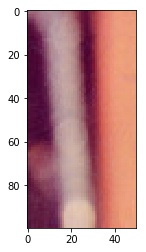### c- Modify the following function so that it can load an RGB image and convert it to a grayscale image using the formula L=0.2989 R + 0.5870 G + 0.1140 * B, where R, G and B are the three color channels of the color image and L is the luminance (i.e. the grayscale image). Include a test so that your function also works if the input image is grayscale.¶

In :
def load_image(name,crop_window=-1):

# if the input is already grayscale,
# i.e. if it's a 2D array, just return it
if I.ndim == 2:
return I
else:
# it's a color image (3D array: there's an additional dimension for the RGB coordinates)
# The following dot product is a weighted sum over the RGB coordinates (last axis) of I,
#  whose weights are the values of [0.2989, 0.5870, 0.1140], which is what we want
# (cf https://docs.scipy.org/doc/numpy/reference/generated/numpy.dot.html)
return np.dot(I, np.array([0.2989, 0.5870, 0.1140]))

plt.imshow(I_cropped_grayscaled)

Out:
<matplotlib.image.AxesImage at 0x1814614160>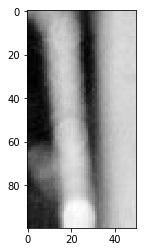Use your function to load a grayscale version of lena and save it.

In :
I = load_image(name)
plt.imshow(I)

Out:
<matplotlib.image.AxesImage at 0x1814ebb898>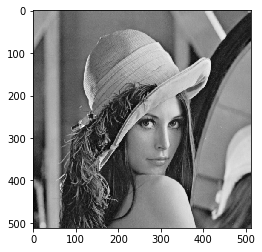### d- Here is an example of how to convolve an image with a Gaussian. What is the size of the output? What are the border conditions? Replace the border conditions with zero padding and comment on the result¶

In :
Ib = ndimage.gaussian_filter(I, sigma=3, order=1)

display(Markdown("> The size of the output **is** {} the same as the input"\
.format("**not**"*(Ib.size != I.size))))

plt.imshow(Ib)


The size of the output is the same as the input

Out:
<matplotlib.image.AxesImage at 0x1815b47748>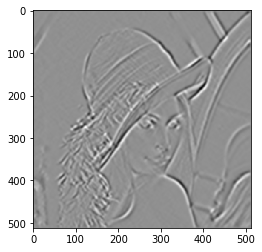1. The output is of the same shape (and size) as the input.
2. The border conditions are determined by the mode paramater, whose default value is reflect. That is, when applying the filter to the borders, the values of the last row/column are reflected across the image's boundary.
3. To replace the border conditions with zero padding, the mode parameter has to be set to constant (constant padding), the constant value (determined by the cval paramater) being 0.0 by default.
In :
Ib = ndimage.gaussian_filter(I, sigma=3, mode='constant')
plt.imshow(Ib)

Out:
<matplotlib.image.AxesImage at 0x181634fcc0>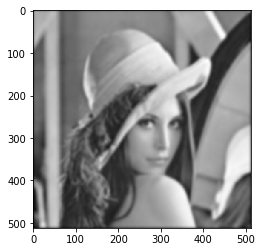The black outline is due to the border conditions being set to zero padding: on the borders, the gaussian window pixels outside the boundary are black (constant value 0), resulting in a blacker weighted average.

### e- What does the following code do?¶

In :
k = np.array([[-1./9, -1./9, -1./9],
[-1./9, 2.-1/9., -1./9],
[-1./9, -1./9, -1./9]])
Is=ndimage.convolve(I, k)
plt.imshow(Is)

Out:
<matplotlib.image.AxesImage at 0x18165fdc18>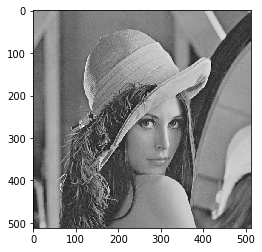As seen in class (page 56), this linear filter sharpens the image (by accentuating the difference with the local average):

dirac_two = np.zeros((3, 3))
dirac_two[1,1] = 2
k = dirac_two - 1./9 * np.ones((3, 3))


$$k = \underbrace{\begin{pmatrix} 0 & 0 & 0 \\ 0 & 2 & 0 \\ 0 & 0 & 0 \\ \end{pmatrix}\;}_{\text{accentuation}} \;\; - \; \underbrace{\begin{pmatrix} \frac 1 9 & \frac 1 9 & \frac 1 9 \\ \frac 1 9 & \frac 1 9 & \frac 1 9 \\ \frac 1 9 & \frac 1 9 & \frac 1 9 \end{pmatrix}\;}_{\text{local average}}$$

### f- Write a function compute_gradient that returns three images containing an image derivative along the horizontal direction, the vertical direction, and the gradient norm. It should use forward differences, and take an optional argument sigma that gives the scale of the derivative. What is the size of the output compared to the size of the input?¶

In :
def compute_gradient(I, sigma=3):
# derivative along horizontal (resp. vertical) direction:
# we use the fact that d/dx(G * f) = d/dx(G) * f (resp. d/dx(G * f) = d/dx(G) * f)
# where G is a gaussian kernel
I_horiz = ndimage.gaussian_filter1d(I, sigma, order=1, axis=1)
I_vert = ndimage.gaussian_filter1d(I, sigma, order=1, axis=0)

# ||grad f|| = sqrt((df/dx)^2 + (df/dy)^2)

display(Markdown("#### {}".format(label)))
plt.imshow(img)
plt.show()
display(Markdown("> The size of the output **is** {} the same as the input\n \n_______"\
.format("**not**"*(img.size != I.size))))


#### Horizontal derivative¶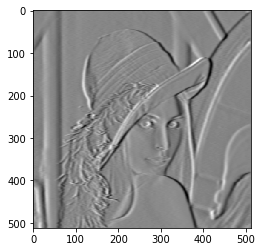The size of the output is the same as the input

#### Vertical derivative¶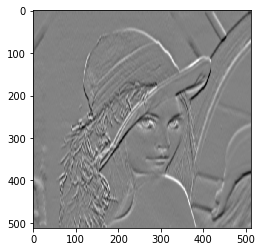The size of the output is the same as the input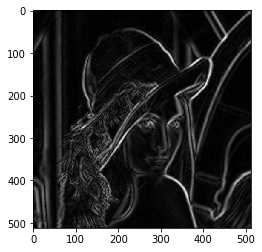The size of the output is the same as the input

### g- Visualize the three images returned by your function for different values of sigma. Comment.¶

Tip: if you want to display several images in a single cell, use plt.show() after each call to plt.imshow(...)

In :
sigma_range = range(1, 5)

for sigma in sigma_range:
display(Markdown("#### sigma = {}".format(sigma)))

fig = plt.figure(figsize = (15., 15.))

# for sigma=sigma, retrieve the images returned by compute_gradient
# and add them to a subplot
for (index, img), label in zip(enumerate(compute_gradient(I, sigma=sigma)), \
["Horizontal derivative", "Vertical derivative", "Gradient norm"]):